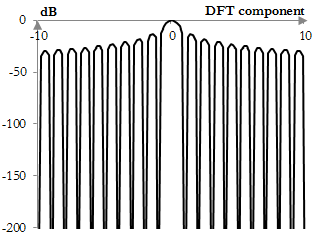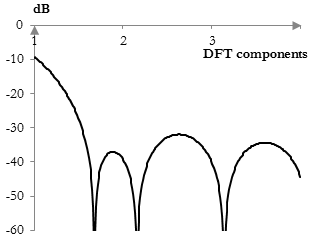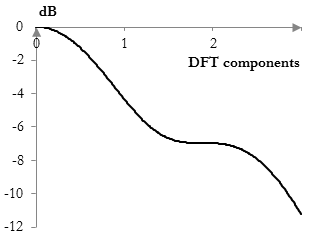# Highest sidelobe level

The highest sidelobe level of a window is the height of the highest lobe (sidelobe, excluding the main lobe) in the Fourier transform of the window.

The following is the middle portion of a 500 point Fourier transform of the rectangular window.Excluding the main lobe in the middle, the highest lobes are the two lobes next to the middle one. Their height is -13.3 dB. Thus, the highest sidelobe level of the rectangular window is approximately -13.3 dB.

## Motivation for the highest sidelobe level measurement

By the convolution theorem, the Fourier transform of the product of two functions is the convolution of the Fourier transforms of the two functions. Thus, applying a window to a filter is equivalent to using the magnitude response of the window as a filter on the magnitude response of the filter. The smaller (larger negative) the highest sidelobe level is, the more likely the Fourier transform of the window will resemble an impulse (depending on the size of the middle lobe), and the more the magnitude response of the window will act on the magnitude response of the filter as an all pass filter, producing less change.

## Examples of the highest sidelobe level

Note that the highest sidelobe is not always the one next to the main lobe. The following figure shows the main lobe and three of the sidelobes of the Gaussian window (σ = 0.5).The highest sidelobe is the second sidelobe, not the one next to the main lobe.

In some cases the term highest sidelobe level is difficult to interpret. As the frequency ω0 of the Dolph-Chebychev window increases, the sidelobes of the discrete Fourier transform of the window are flattened out slowly, and the highest sidelobe becomes the next one. The following shows a sidelobe of an example Dolph-Chebychev window (ω0 = 0.2).## Highest sidelobe level for common windows

The following is the highest sidelobe level of some common windows (in dB, with the window definitions on this site).

 Bartlett-Hann -35.9 BlackmanExact BlackmanGeneralized Blackman  α = 0.05  α = 0.20  α = 0.35 -58.1-68.7 -37.3-56.5-38.1 Blackman-Harris -92.0 Blackman-Nuttall -98.3 Bohman -46.0 Dolph-Chebychev  ω0 = 0.1  ω0 = 0.2  ω0 = 0.3 -29.6-31.9-35.1 Flat top -93.6 Gaussian  σ = 0.3  σ = 0.5  σ = 0.7Approximate confined Gaussian  σ = 0.3  σ = 0.5  σ = 0.7Generalized normal  α = 2  α = 4  α = 6 -64.3-32.0-20.9 -63.6-30.3-23.5 -32.0-19.7-16.3 Hamming -44.4 Hann -31.5 Hann-Poisson  α = 0.3  α = 0.5  α = 0.7 -37.6-35.2-33.0 Kaiser  α = 0.5  α = 1.0  α = 5.0 -16.6-24.6-38.6 Kaiser-Bessel -65.3 Lanczos -26.4 Nuttall -93.3 Parzen -24.0 Planck-taper  ε = 0.2  ε = 0.4   ε = 0.5 -13.9-17.5-23.2 Poisson  α = 0.2  α = 0.5  α = 0.8 -14.7-17.5-19.9 Power of cosine  α = 1.0  α = 2.0  α = 3.0 -23.0-31.5-39.3 Rectangular -13.3 Sine -23.0 Triangular -26.5 Tukey  α = 0.3  α = 0.5  α = 0.7 -18.2-15.1-13.8 Ultraspherical (x0 = 1)  μ = 2  μ = 3  μ = 4 -21.3-27.7-33.3 Welch -21.3

### Filtered HTML

• Freelinking helps you easily create HTML links. Links take the form of [[indicator:target|Title]]. By default (no indicator): Click to view a local node.